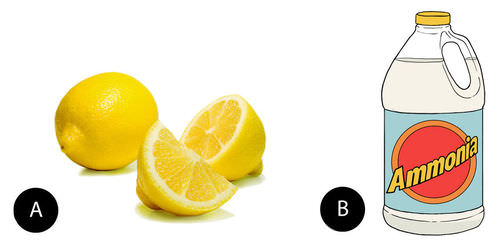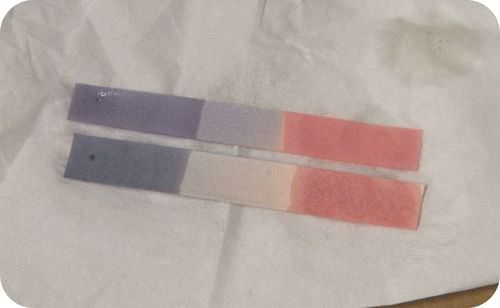# 4.13: Arrhenius Acids and Bases

Learning Outcomes

• Understand that all Arrhenius Acids produce H+ or protons in water.
• Understand that all Arrhenius Bases produce OH- or hydroxide in water.
• Write formulas for binary and ternary Arrhenius Acids.
• Write formulas for Arrhenius Bases.
• Appreciate the pH scale.
• Know applications of selected Arrhenius Acids and Bases.
• Describe the properties of acids and bases.

## pH ScaleFigure $$\PageIndex{0}$$: Average pH value of seawater in 1870 and 2100 (estimated). The difference may seem small, but the decline in the pH value from 1970 to 2100 would mean a 170 % increase in the acidity. Much small changes already post problems fro many sea creatures. pH value of various substances (Hydrochloric acid, Cola, Sopa, Laundry detergent, and more). (CC BY-SA 2.0; Generic; Heinrich-Böll-Stiftung).

## Arrhenius Definitions

The Swedish chemist Svante Arrhenius (1859 - 1927) was the first to propose a theory to explain the observed behavior of acids and bases. Because of their ability to conduct a current, he knew that both acids and bases contained ions in solution. An Arrhenius acid is a compound which ionizes to yield hydrogen ions $$\left( \ce{H^+} \right)$$ in aqueous solution. An Arrhenius base is a compound which ionizes to yield hydroxide ions $$\left( \ce{OH^-} \right)$$ in aqueous solution.

## Acid Properties

Acids are very common in some of the foods that we eat. Citrus fruits such as oranges and lemons contain citric acid and ascorbic acid, which is better known as vitamin C (see figure below). All carbonated beverages contain carbonic acid. This chemical easily decomposes to produce bubbly carbon dioxide and water. Acids can be commonly found in many places. For example, your stomach uses gastric acid (hydrochloric) to dissolve food. Vinegar is a mixture of water and acetic acid. This solution preserves condiments like ketchup and barbeque sauce.Figure $$\PageIndex{1}$$: (A) Lemons and other citrus fruits contain citric and ascorbic acids. (B) Ammonia is a base that is present in many household cleaners.

Acids are a distinct class of compounds because of the properties of their aqueous solutions. Those properties are outlined below.

1. Aqueous solutions of acids are electrolytes, meaning that they conduct an electrical current. Some acids are strong electrolytes because they ionize completely in water. Other acids are weak electrolytes which partially ionize when dissolved in water.
2. Acids have a sour taste. Lemons, vinegar, and sour candies all contain acids.
3. Acids change the color of certain acid-base indicators .Two common indicators are litmus and phenolphthalein. Blue litmus turns red in the presence of an acid, while phenolphthalein remains colorless.
4. Acids react with some metals to yield hydrogen gas.
5. Acids (pH < 7.0) react with bases (pH > 7.0) to produce a salt and water. When equal moles of an acid and a base are combined, the acid is neutralized by the base. The resulting mixture will have a more neutral pH.

### Formula writing and Applications of Arrhenius Acids

If the acid name begins with the prefix " hydro" then it is a binary acid. These types of acids involve anions from the periodic table. For " hydro" acids, perform the steps below:

1. Start the formula with a proton (H+) and refer to the periodic table for the anion species.
2. Once charges from step #1 have been obtained, cross the charges down diagonally and remove signs (+/-)
3. Acid formulas will need to display the state of matter (aq) beside the chemical formula.

If the acid name does not begin with the prefix "hydro," then it is a polyatomic or ternary acid.

1. Start the fomula with a proton (H+) and note the ending of the acid name. Acids that have "ic" endings will use the "ate" polyatomic ion. In contrast, acids that have "ous" endings will use the "ite" polyatomic ion.
2. Cross the proton and polyatomic charges down and remove signs (+/-).
3. Acid formulas will need to display the state of matter (aq) beside the chemical formula.
Table $$\PageIndex{1}$$: Common Acids
Acid Name Formula Real-world Applications
hydrochloric acid $$\ce{HCl}$$ Contained in stomach acid and liquid toliet bowl cleaner
nitric acid $$\ce{HNO_3}$$ Contained in chemical bombs and fertilizers.
sulfuric acid $$\ce{H_2SO_4}$$ Wet batter acid, chemical bomb, and fertilizers.
phosphoric acid $$\ce{H_3PO_4}$$ Preservative in dark colored colas.
acetic acid $$\ce{CH_3COOH}$$ Active ingredient of vinegar solution
hypochlorous acid $$\ce{HClO}$$ Acid used to sanitize water.
carbonic acid $$\ce{H_2CO_3}$$ Acid contained in all carbonated beverages

A monoprotic acid is an acid that contains only one ionizable hydrogen. Hydrochloric acid and acetic acid are monoprotic acids. A polyprotic acid is an acid that contains multiple ionizable hydrogens. Most common polyprotic acids are either diprotic (such as $$\ce{H_2SO_4}$$), or triprotic (such as $$\ce{H_3PO_4}$$).

### Bases Properties

Bases have properties that mostly contrast with those of acids.

1. Arrhenius bases that are soluble in water can conduct electricity.
2. Bases often have a bitter taste and are found in foods less frequently than acids. Many bases, like soaps, are slippery to the touch.
3. Bases also change the color of indicators. Red litmus turns blue in the presence of a base (see figure below), while phenolphthalein turns pink.
4. Some bases react with metals to produce hydrogen gas.
5. Acids (pH < 7.0) react with bases (pH > 7.0) to produce a salt and water. When equal moles of an acid and a base are combined, the acid is neutralized by the base. The resulting mixture will have a more neutral pH.Figure $$\PageIndex{2}$$: Litmus paper has be treated with the plant dye called litmus. It turns red in the presence of an acid and blue in the presence of a base.

### Applications of Arrhenius Bases

Bases are ionic compounds which yield the hydroxide ion $$\left( \ce{OH^-} \right)$$ upon dissociating in water. The table below lists several of the more common bases.

Table $$\PageIndex{2}$$: Common Bases
Base Name Formula Real-world Applications
Sodium hydroxide $$\ce{NaOHDra Potassium hydroxide \(\ce{KOH}$$
Magnesium hydroxide $$\ce{Mg(OH)_2}$$
Calcium hydroxide $$\ce{Ca(OH)_2}$$
Ammonium hydroxide $$\ce{NH_4OH}$$

All of the bases listed in the table are solids at room temperature. Upon dissolving in water, each dissociates into a metal cation and the hydroxide ion.

$\ce{NaOH} \left( s \right) \overset{\ce{H_2O}}{\rightarrow} \ce{Na^+} \left( aq \right) + \ce{OH^-} \left( aq \right)$

Sodium hydroxide is a very caustic substance also known as lye. Lye is used as a rigorous cleaner and is an ingredient in the manufacture of soaps. Care must be taken with strong bases like sodium hydroxide, as exposure can lead to severe burns (see figure below).Figure $$\PageIndex{4}$$: This foot has severe burns due to prolonged contact with a solution of sodium hydroxide, also known as lye.

## Brønsted-Lowry Acids and Bases

The Arrhenius definition of acids and bases is somewhat limited. There are some compounds whose properties suggest that they are either acidic or basic, but which do not qualify according to the Arrhenius definition. An example is ammonia $$\left( \ce{NH_3} \right)$$. An aqueous solution of ammonia turns litmus blue, reacts with acids, and displays various other properties that are common for bases. However, it does not contain the hydroxide ion. In 1923, a broader definition of acids and bases was independently proposed by the Danish chemist Johannes Brønsted (1879 - 1947) and the English chemist Thomas Lowry (1874 - 1936). A Brønsted-Lowry acid is a molecule or ion that donates a hydrogen ion in a reaction. A Brønsted-Lowry base is a molecule or ion that accepts a hydrogen ion in a reaction. Because the most common isotope of hydrogen consists of a single proton and a single electron, a hydrogen ion (in which the single electron has been removed) is commonly referred to as a proton. As a result, acids and bases are often called proton donors and proton acceptors, respectively, according to the Brønsted-Lowry definition. All substances that are categorized as acids and bases under the Arrhenius definition are also define as such under the Brønsted-Lowry definition. The new definition, however, includes some substances that are left out according to the Arrhenius definition.